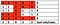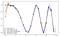# Extracting image features without Deep Learning

Image is a matrix of numbers, let’s convert the knowledge into features.

Introduction:

One of the hottest fields in data science is image classification, in this article I want to share some techniques for transforming image to a vector of features that can be used in every classification model after then.

As a data scientist in VATbox I usually work with texts or images, in this article I’ll combine them both and we will try to solve text problem using only image.

Problem definition:

VATbox, as the name suggests, deals with VAT problems (and a lot more) one of the problems in the invoices world is that I would want to know how many invoices are in one image? To simplify the question, we will ask a binary question, do we have one invoice in the image or multiple invoices in the same image?

Why not use the text (TF-IDF for example)? Why use only the image pixels as an input?

So, sometimes we don’t have a reliable OCR, sometimes the OCR costs us money and we are not sure we want to use it..and for this article of course, to demonstrate the power of classical approaches for extracting features from an image.

Some python code for getting started:

`import cv2gray_image = cv2.imread(image_path, 0)img = image.load_img(image_path, target_size=(self.IMG_SIZE, self.IMG_SIZE))`

image reduction:

imagine that you are staring at the image very closely, you can see the pixels up close..so if we have image that contains text we can see the white pixels between the words and between the rows. If our intention (at least in this case) is to decide if we have a single invoice in the image, we can look on the image from some distance — it will help the “boring” white spaces in the image to be neglected.

`# scale parameter – the relative size of the reduced image after the reduction.image_width = int(gray_image.shape * scale_percent)image_height = int(gray_image.shape * scale_percent)dim = (width, height)gray_reduced_image = cv2.resize(gray_image, dim, interpolation=cv2.INTER_NEAREST)cv2.imshow('image', resized)cv2.waitKey(0)`

So..which features we are going to use?

Image Entropy:

we can think about it like this — the difference between multiple invoices or single invoice per image can be translated to the amount of information in the image, thus, we can expect different mean entropy score in each class.

Where n is the sum number of gray levels (256 for 8-bit images) and p is the probability of a pixel having gray level i.

`from sklearn.metrics.cluster import entropyentropy1 = entropy(gray_image)entropy2 = entropy(gray_reduced_image)`

DBscan:

Dbscan algorithm has the ability to find dense areas in our image space and assign them to one cluster. Its biggest advantage is that it determines the number of classes in the data by itself. We will create 3 features from the dbscan model:

1. number of classes (the assumption here is that a high number of classes will indicate a multiplicity of invoices in the image).
`from sklearn.cluster import DBSCANfrom sklearn import metricsthr, imgage = cv2.threshold(gray_reduced_image, 0, 255, cv2.THRESH_BINARY + cv2.THRESH_OTSU)img_df = pd.DataFrame(img).unstack().reset_index().rename(columns={'level_0': 'y', 'level_1': 'x'})img_df = img_df[img_df == 0]X = image_df[['y', 'x']]db = DBSCAN(eps=1, min_samples=5).fit(X)# plt.scatter(image_df['y'], image_df['x'], c=db.labels_, s=3)# plt.show(block=False)core_samples_mask = np.zeros_like(db.labels_, dtype=bool)core_samples_mask[db.core_sample_indices_] = Truelabels = db.labels_# Number of clusters in labels, ignoring noise if present.n_clusters_ = len(set(labels)) - (1 if -1 in labels else 0)n_noise_ = list(labels).count(-1)image_df['class'] = labels# print('Estimated number of clusters: %d' % n_clusters_)# print('Estimated number of noise points: %d' % n_noise_)# print("Silhouette Coefficient: %0.3f" % metrics.silhouette_score(image_df, labels))features = pd.Series([n_clusters_, n_noise_, metrics.silhouette_score(image_df, labels)])`

Compute zero crossings:

Each pixel in our (gray scale) image has a value between 0–255 (in our case zero is taken to be white, and 255 is taken to be black). If we want to compute “zero” crossings we’ll need to threshold the image — i.e. set a value such that a higher value will classify as 255 (black) and lower value will classify as 0 (white). In our case I used Otsu thresholding. After we will perform image thresholding, we will get zeros and ones as pixels, we can look at this as dataframe and sum each column and each row:Example rows/cols pixels summing, it’s easy to see that the sum line creates an useful histogram for our usage.

Now, imagine that the 1’s stands for areas with text (black pixels) and the 0’s for blanks (white pixels). We now can count the numbers of times that each row/col sum changes from any positive number to zero.

`img = img / 255df = pd.DataFrame(img)pixels_sum_dim1 = (1 - img_df).sum()pixels_sum_dim2 = (1 - img_df).T.sum()zero_corssings1 = pixels_sum_dim1[pixels_sum_dim1 == 0].reset_index()['index'].rolling(2).apply(np.diff).dropna()zero_corssings1 = zero_corssings1[zero_corssings1 != 1]num_zero1 = zero_corssings1.shapezero_corssings2 = pixels_sum_dim2[pixels_sum_dim2 == 0].reset_index()['index'].rolling(2).apply(np.diff).dropna()zero_corssings2 = zero_corssings2[zero_corssings2 != 1]num_zero2 = zero_corssings2.shapefeatures = pd.Series([num_zero1, num_zero2])`

Normalized image histogram:

If we will treat our image as a signal, we can use some tools from the signal processing toolbox. We will use the re-sampling idea to create some more features.

How to do so? First, we need to convert our image from matrix to one dimensional vector. Second, since each image has a different shape we need to set one re-sampling size for all the images — in our case I used 16, what is it mean?

Using interpolation we can represent the signal as a continues function and then we will re-sample from it, the spacing between samples is

where x denotes the image signal and C denotes the number of points to resample.in this example you can find plot of several resampling methods of the function f(x) = cos(-x²/6)
`from scipy.signal import resampledim1_normalized_hist = pd.Series(resample(df.sum(), 16))dim2_normalized_hist = pd.Series(resample(df.T.sum(), 16))print(dim1_normalized_hist)print(dim2_normalized_hist)`

DCT — Discrete Cosine Transform:

A discrete cosine transform (DCT) expresses a finite sequence of data points in terms of a sum of cosine functions oscillating at different frequencies. DCT unlike DFT (Discrete Fourier Transform) has only real part.

The DCT, and in particular the DCT-II, is often used in signal and image processing, especially for lossy compression, because it has a strong “energy compaction” property. In typical applications, most of the signal information tends to be concentrated in a few low-frequency components of the DCT.

We can compute the DCT vector on the image and on the transpose image, and take the first k elements.

`from scipy.fftpack import dctdim1_dct = pd.Series(dct(df.sum())[0:8]).to_frame().Tdim2_dct = pd.Series(dct(df.T.sum())[0:8]).to_frame().Tdim1_normalize_dct = pd.Series(normalize(dim1_dct).tolist())dim2_normalize_dct = pd.Series(normalize(dim2_dct).tolist())print(dim1_normalize_dct)print(dim2_normalize_dct)`

Conclusion

These days the use of CNN’s is growing, in this article I tried to explain and demonstrate some classic ways to create features from the image in the old fashioned way, it would be good practice to know the basic of image processing because some times it easier and more accurate than just pushing it into a net. This article is an introduction and maybe a brain stimulation for what we can do with images and how we can use and extract knowledge from the pixels.

data scientist & team leader, NLP researcher

## More from Yuval Cohen

data scientist & team leader, NLP researcher